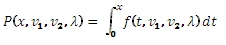# SQL Server non-central F inverse distribution function

NCFINV

Updated: 31 July 2010

Use NCFCDF to calculate the inverse of the lower cumulative distribution function of the non-central F distribution.

The formula for the lower cumulative density function is:Syntax
SELECT [wctStatistics].[wct].[NCFINV] (
<@CDF, float,>
,<@DF1, float,>
,<@DF2, float,>
,<@Lambda, float,>)
Arguments
@CDF
is cumulative distribution function. @CDF is an expression of type float or of a type that implicitly converts to float
@DF1
degrees of freedom. @DF1 is an expression of type float or of a type that implicitly converts to float
@DF2
degrees of freedom. @DF2 is an expression of type float or of a type that implicitly converts to float
@Lambda
is the non-centrality parameter. @Lambda is an expression of type float or of a type that implicitly converts to float
Return Types
float
Remarks
·         @CDF must be greater than zero and less than one (0 < @CDF < 1).
·         @DF1 must be greater than zero (@DF1 > 0).
·         @DF2 must be greater than zero (@DF2 > 0).
·         @Lambda must be greater than or equal to zero (@Lambda > 0).
Examples
Calculate the lower cumultive distribution function:
SELECT wct.NCFCDF(0.5,3,2,1)
This produces the following result
----------------------
0.210838872156613

(1 row(s) affected)

Calculate the inverse:
SELECT wct.NCFINV(0.210838872156613,3,2,1)
This produces the following result
----------------------
0.5

(1 row(s) affected)

### SupportCopyright 2008-2021 Westclintech LLC         Privacy Policy        Terms of Service